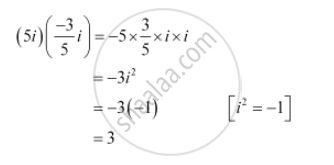# Express the given complex number in the form a + ib: (5i)(-35i) - Mathematics

Sum

Express the given complex number in the form a + ib: (5i) (- 3/5 i)

#### SolutionConcept: Concept of Complex Numbers
Is there an error in this question or solution?
Chapter 5: Complex Numbers and Quadratic Equations - Exercise 5.1 [Page 103]

#### APPEARS IN

NCERT Class 11 Mathematics
Chapter 5 Complex Numbers and Quadratic Equations
Exercise 5.1 | Q 1 | Page 103

Share